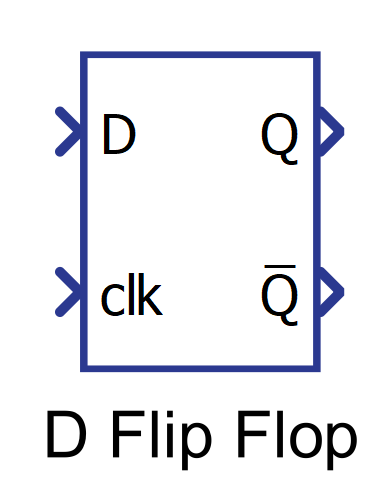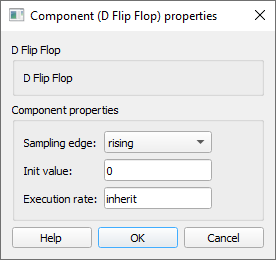# D Flip Flop

Description of the D Flip Flop component in Schematic Editor, which tracks the Boolean value on its input and updates its output according to the clock signal.

## Component Icon## Description

The D Flip Flop component captures the value of the D-input at the rising/ falling edge of the clock signal and outputs it on the Q terminal.

Every time a rising/falling edge is detected on the clock signal, the Flip Flop samples the D input value and stores it. That value is persistent on the Q output until the next clock edge is detected, when the new value is sampled and stored.

You can choose if the sampling is done on the rising or falling clock edge using the Sampling edge property.

The D Flip Flop component stores the value as Boolean. Every number that is not 0 will be treated as 1.

The output is the inverted state output.

The output of D Flip Flop can be described using the truth table of Table 1 where X denotes a “don’t care” position, which means that the input signal is irrelevant:

 D Clock Q Q̅ 0 edge (↑ or ↓) 0 1 1 edge (↑ or ↓) 1 0 X No edge No change No change

## Ports

• Input D (in)
• Sampled input.
• Supported types: uint, int and real.
• Vector support: yes.
• Clock clk (in)
• Clock signal.
• Supported types: uint, int and real.
• Vector support: yes.
• Output Q (out)
• This is the output of the component, in accordance with the Truth table.
• Supported types: uint, int and real.
• The output type is inherited from the input signal.
• Vector support: yes.
• The vector length is inherited from the input signal or calculated using property value lengths. If the property is defined as a vector, the output will be a vector of the same length.
• Output Q̅ (out)
• This is the inverse of the output Q, according with the Truth table.
• Supported types: uint, int and real.
• The output type is inherited from the input signal.
• Vector support: yes.
• The vector length is inherited from the input signal or calculated using property value lengths. If the property is defined as a vector, the output will be a vector of the same length.

## Properties• Sampling edge
• Select the edge of the clock signal that will be used for sampling.
• Initial Value
• Type in the initial value of the D Flip Flop component. The property can be scalar or vector.
• Execution rate
• Type in the desired signal processing execution rate. This value must be compatible with other signal processing components of the same circuit: the value must be a multiple of the fastest execution rate in the circuit. There can be up to four different execution rates, but they must all be multiple of the basic simulation timestep. To specify the execution rate, you can use either decimal (e.g. 0.001) or exponential values (e.g. 1e-3) in seconds. Alternatively, you can type in ‘inherit’ in which case the component will be assigned execution rate based on the execution rate of the components it is receiving input from.